# RD Sharma Solutions for Class 9 Maths Chapter 10 Congruent Triangles Exercise 10.3

RD Sharma class 9 Mathematics chapter 10 exercise 10.3 Congruent Triangles solutions are provided here. In this exercise, students will learn whether two given geometrical shapes can be congruent or not. In brief, geometrical figures with the same size and shape are known as congruent figures and the properties known as congruence. The solutions for RD Sharma class 9 chapter 10 will help the students to learn more about congruent triangles.

## Download PDF of RD Sharma Solutions for Class 9 Maths Chapter 10 Congruent Triangles Exercise 10.3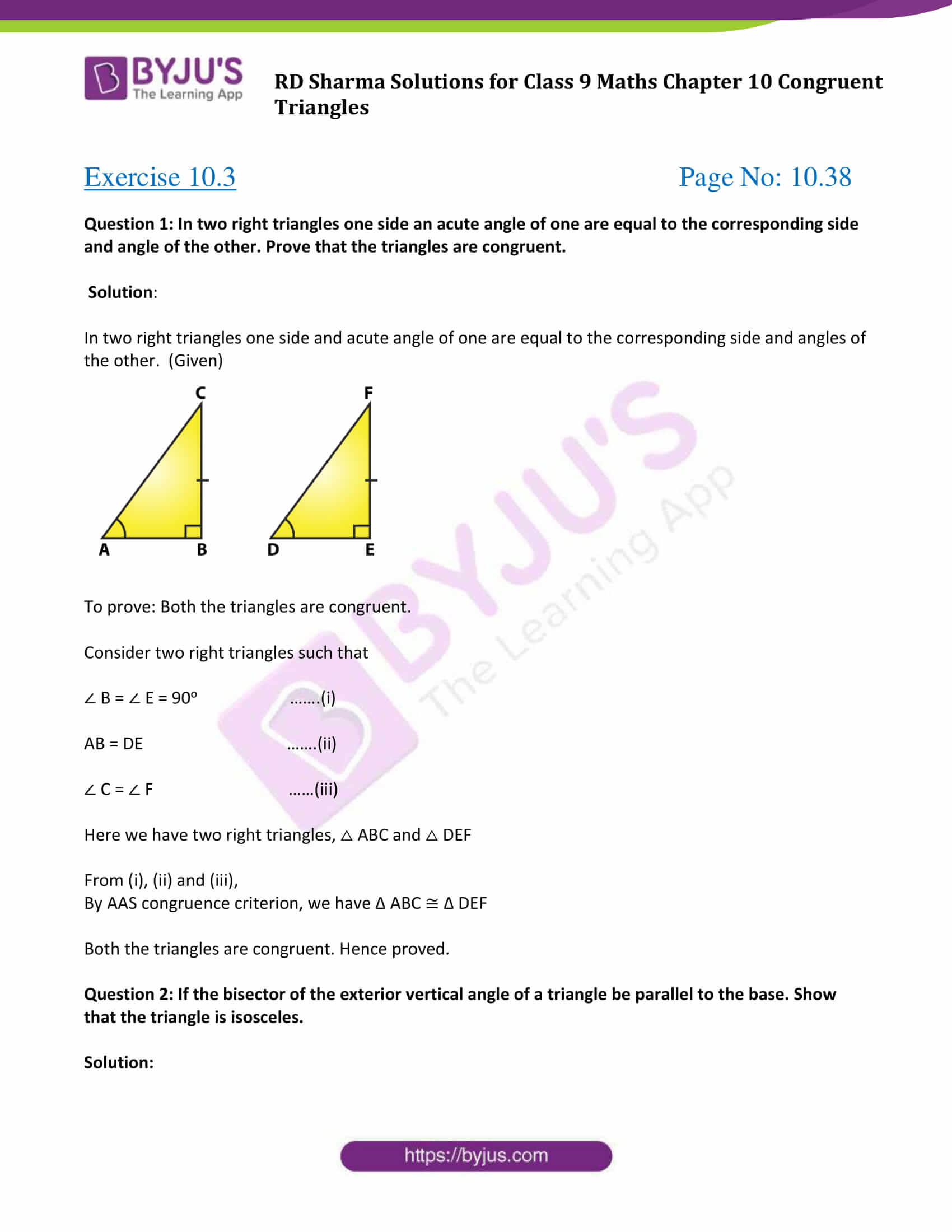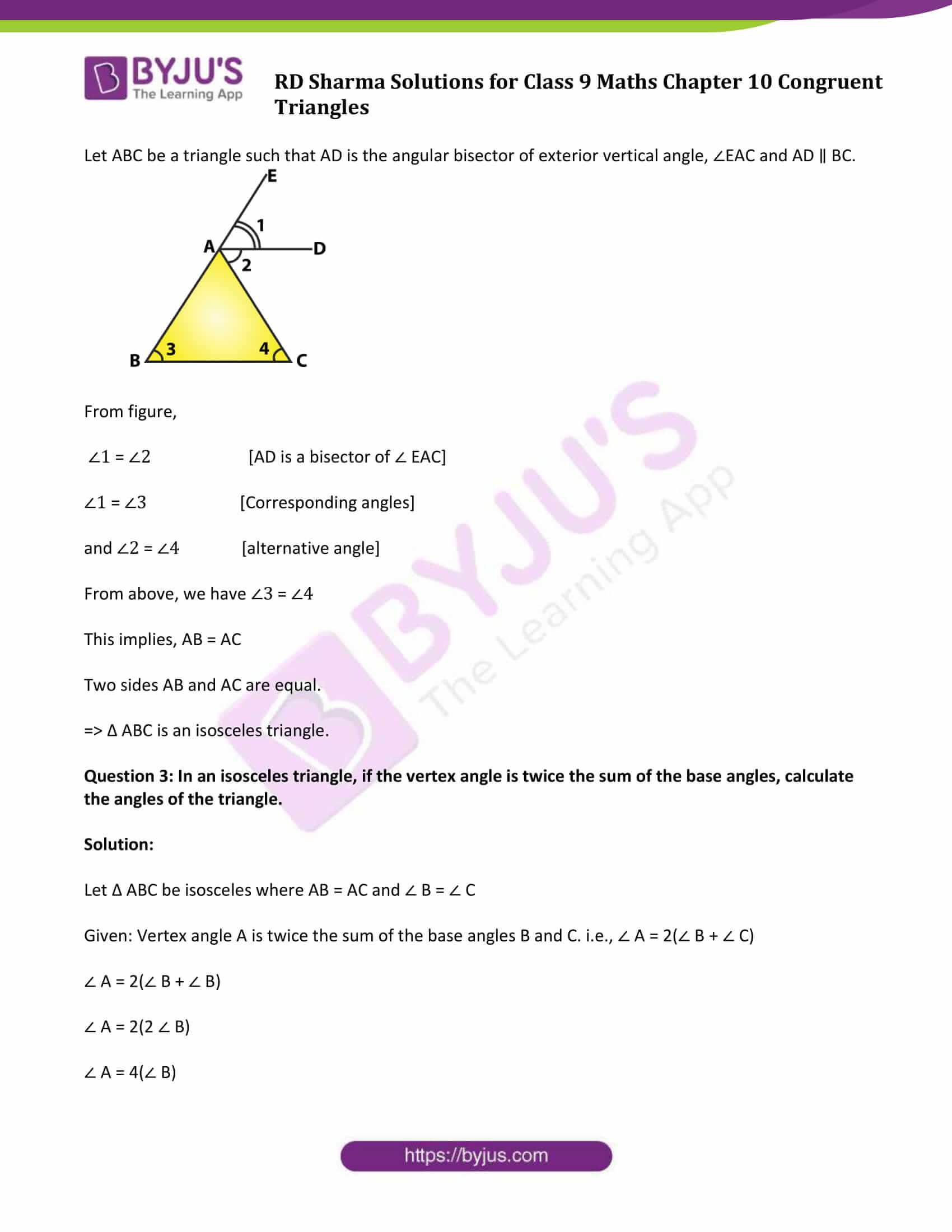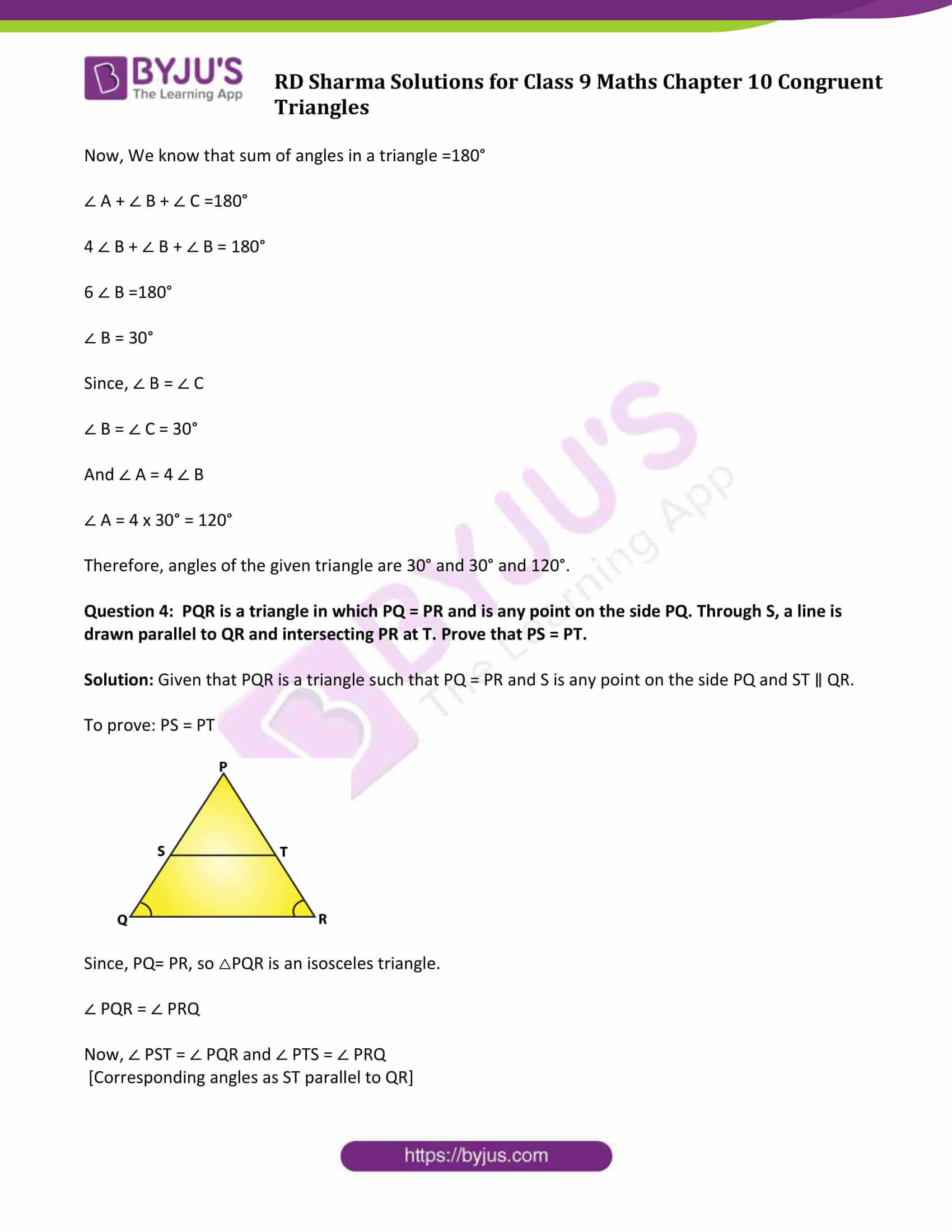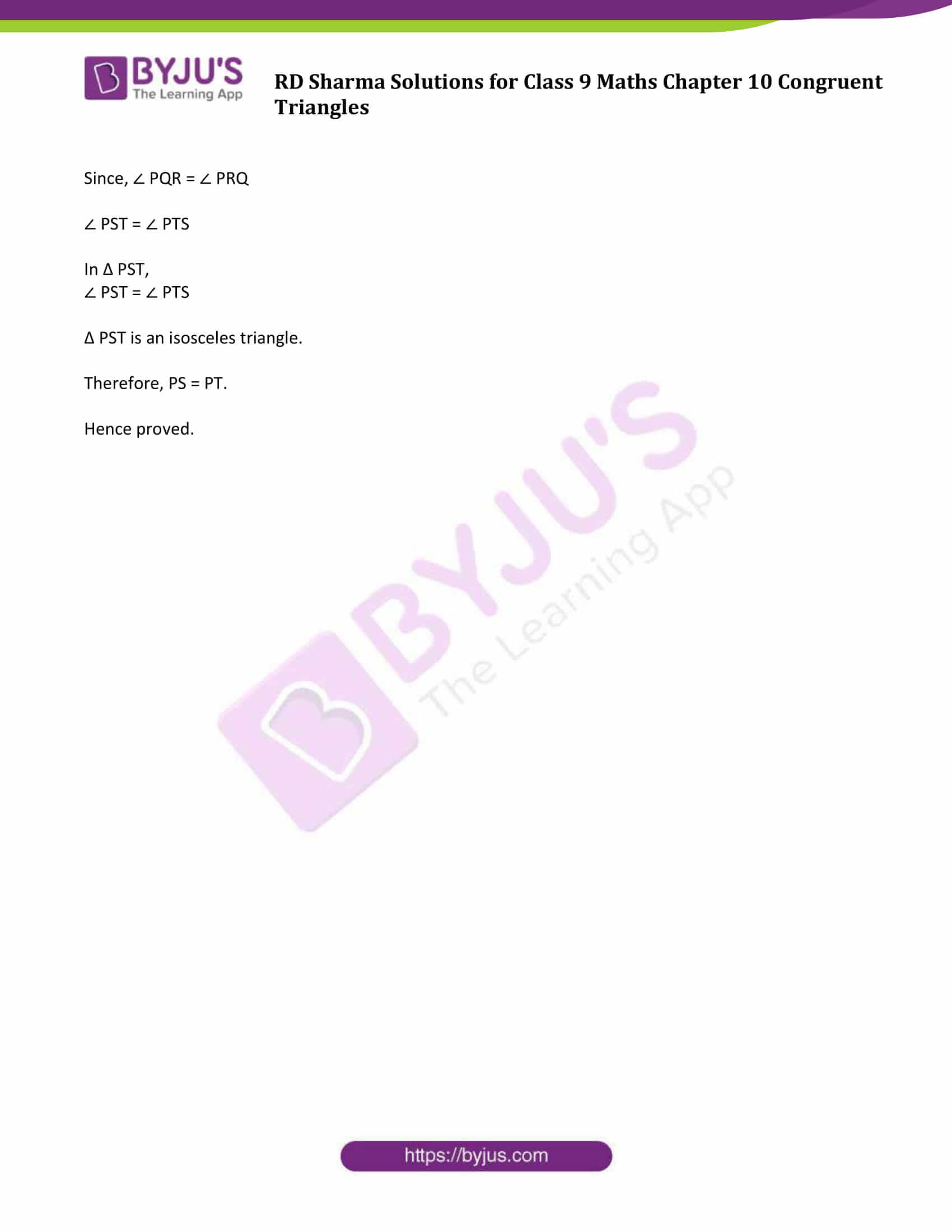### Access Answers to Maths RD Sharma Solutions for Class 9 Chapter 10 Congruent Triangles Exercise 10.3 Page number 10.38

Question 1: In two right triangles one side an acute angle of one are equal to the corresponding side and angle of the other. Prove that the triangles are congruent.

Solution:

In two right triangles one side and acute angle of one are equal to the corresponding side and angles of the other. (Given)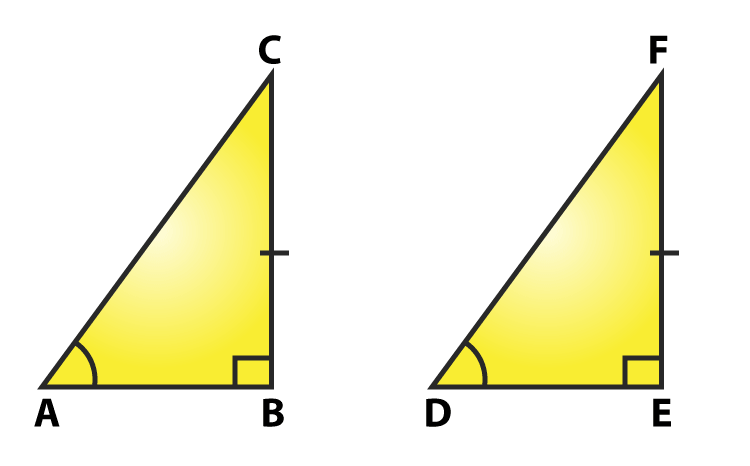To prove: Both the triangles are congruent.

Consider two right triangles such that

∠ B = ∠ E = 90o …….(i)

AB = DE …….(ii)

∠ C = ∠ F ……(iii)

Here we have two right triangles, △ ABC and △ DEF

From (i), (ii) and (iii),

By AAS congruence criterion, we have Δ ABC ≅ Δ DEF

Both the triangles are congruent. Hence proved.

Question 2: If the bisector of the exterior vertical angle of a triangle be parallel to the base. Show that the triangle is isosceles.

Solution:

Let ABC be a triangle such that AD is the angular bisector of exterior vertical angle, ∠EAC and AD ∥ BC.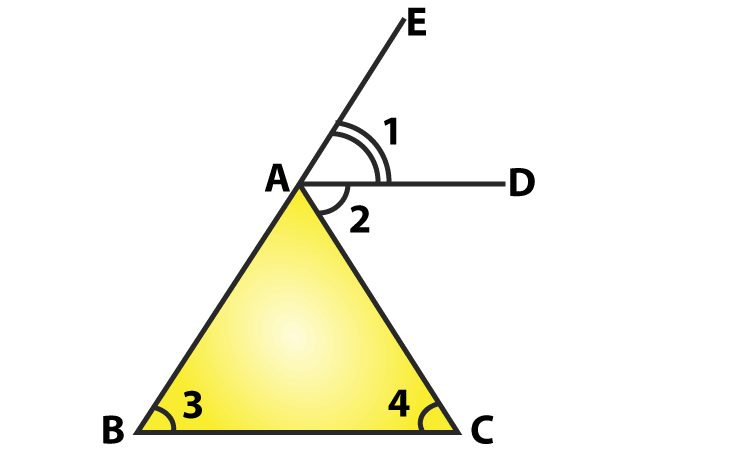From figure,

∠1 = ∠2 [AD is a bisector of ∠ EAC]

∠1 = ∠3 [Corresponding angles]

and ∠2 = ∠4 [alternative angle]

From above, we have ∠3 = ∠4

This implies, AB = AC

Two sides AB and AC are equal.

⇒ Δ ABC is an isosceles triangle.

Question 3: In an isosceles triangle, if the vertex angle is twice the sum of the base angles, calculate the angles of the triangle.

Solution:

Let Δ ABC be isosceles where AB = AC and ∠ B = ∠ C

Given: Vertex angle A is twice the sum of the base angles B and C. i.e., ∠ A = 2(∠ B + ∠ C)

∠ A = 2(∠ B + ∠ B)

∠ A = 2(2 ∠ B)

∠ A = 4(∠ B)

Now, We know that sum of angles in a triangle =180°

∠ A + ∠ B + ∠ C =180°

4 ∠ B + ∠ B + ∠ B = 180°

6 ∠ B =180°

∠ B = 30°

Since, ∠ B = ∠ C

∠ B = ∠ C = 30°

And ∠ A = 4 ∠ B

∠ A = 4 x 30° = 120°

Therefore, angles of the given triangle are 30° and 30° and 120°.

Question 4: PQR is a triangle in which PQ = PR and is any point on the side PQ. Through S, a line is drawn parallel to QR and intersecting PR at T. Prove that PS = PT.

Solution: Given that PQR is a triangle such that PQ = PR and S is any point on the side PQ and ST ∥ QR.

To prove: PS = PT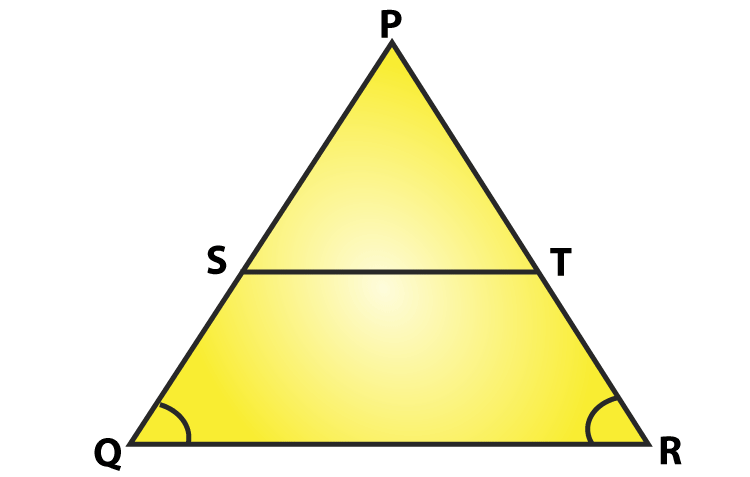Since, PQ= PR, so △PQR is an isosceles triangle.

∠ PQR = ∠ PRQ

Now, ∠ PST = ∠ PQR and ∠ PTS = ∠ PRQ

[Corresponding angles as ST parallel to QR]

Since, ∠ PQR = ∠ PRQ

∠ PST = ∠ PTS

In Δ PST,

∠ PST = ∠ PTS

Δ PST is an isosceles triangle.

Therefore, PS = PT.

Hence proved.

## RD Sharma Solutions for Class 9 Maths Chapter 10 Congruent Triangles Exercise 10.3

RD Sharma Solutions Class 9 Maths Chapter 10 Congruent Triangles Exercise 10.3 is based on the topic- Criterion of Congruence – SAS (Side-Angle-Side). According to the criteria, if two sides and an interior angle of a triangle is equal to the corresponding sides and an interior angle of the other triangle, then both triangles are said to be congruent.